"Fractions are difficult for children to grasp, in part because they represent relative quantities rather than fixed amounts. (Nunes, 2006) Which is bigger: half of a small pizza or a quarter of a large pizza? When considering the size of a fraction, students need to ask, "A fraction of what?" In other words, the size of the whole needs to be defined.

Fractions Made Easy, a new resource by educators Jim Callahan and Marilyn Varricchio, combines teacher-student dialogs, use of visual models, and much guided and individual practice to make fractions ""easy"" for students to understand. The book is organized into three sections, each section covering one of the three Fraction standards laid out in the Common Core State Standards (CCSS) for Grade 3.

Key to the authors' approach is the use of visual models, as called for in the CCSS introduction to Grade 3 math standards: "Students view fractions in general as being built out of unit fractions, and they use fractions along with visual fraction models to represent parts of a whole." (p. 21)

The authors focus on the following visual models:

## Area Models

In third grade, students are introduced to area as a geometric concept, so it makes sense to visualize fractions in terms of area.The authors define ""area models"" as two-dimensional fraction models, usually shown on paper. Examples of area models are fraction bars, fraction strips, and fraction circles.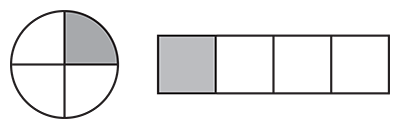The area model is a great visual model for developing a basic understanding of the unit fraction, as outlined in CCSS standard 3.NF.1: Understand a fraction 1/b as the quantity formed by 1 part when a whole is partitioned into b equal parts; understand a fraction a/b as the quantity formed by a parts of size 1/b.

Using fraction rectangles and fraction circles, it is easy to visualize, for example, that 3/4 is comprised of three unit fractions, each the size of 1/4, and that 1/4 is a single part of the whole, 4/4.

Once students have grasped the basic idea of the unit fraction, they should feel comfortable enough to begin exploring fraction concepts using fraction circles (discrete models) and number lines (linear models).

## Linear Models

The number line is another powerful means of visualizing fractions. A number line divided into equal parts between 0 and 1 reinforces the concept of the unit fraction and equal parts making a whole.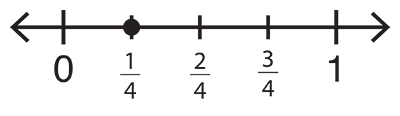Authors Callahan and Varricchio remind us, however, that the number line is inherently difficult for some students to learn. Teachers should discuss with students the meaning of "intervals" and "endpoints" on the number line, pointing out that an interval is the distance between any two endpoints. Thus a unit fraction is represented by an interval with adjacent endpoints. The whole is a larger interval that also has endpoints, labeled as 0 and 1, as this diagram shows: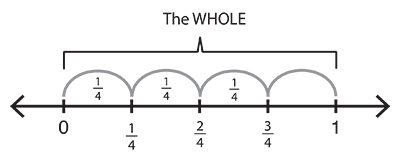Despite the potential for confusion, the CCSS sees fit to dedicate an entire fraction standard to the number line:

3.NF.2. Understand a fraction as a number on the number line; represent fractions on a number line diagram.

Number lines are a great tool for not only reinforcing the idea of unit fractions as parts relating to a whole, but also the idea of fraction equivalence. When two number lines of equal length representing equal wholes are lined up, it is easy to visualize that 1/2 is the same size as 3/6: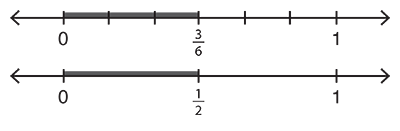## Discrete Models

Discrete models, as defined by the authors, are three-dimensional manipulatives, such as fraction circles and fraction squares, in which the fraction pieces can be physically separated from the whole.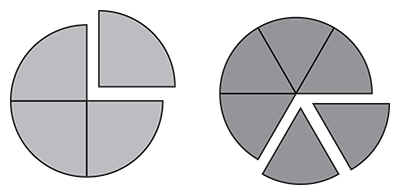This allows students to compare the relative size of fractions by physically stacking the parts, or unit fractions, one on top of another. The individual parts can be removed from the whole circle or combined to make a whole circle.

Fraction circles' stackability makes it easy to demonstrate the relative size of different fractions in relation to the whole (the entire fraction circle). For example, students can see that 1/2 and 2/4 of the same whole are equivalent by placing one fraction on top of the other. They can also see that 2/6 and 3/8 are not equivalent, simply by placing 2/6 on top of 3/8 and seeing that part of 3/8 remains visible.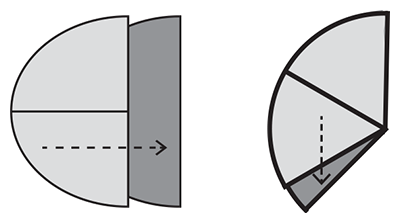Thus, fraction circles, like the number line, are ideal for introducing the concept of fraction equivalence:

3.NF.3: Explain equivalence of fractions in special cases, and compare fractions by reasoning about their size.

Both number lines and fraction circles make it easy to compare fractions with the same numerator (1/4 and 1/6) or same denominator (3/8 and 5/8). As students compare different fractions and reason about their size, they learn to use the symbols =, >, and < to record these comparisons, meeting standard 3.NF.3d: Record the results of comparisons with the symbols >, =, or <, and justify the conclusions, e.g., by using a visual fraction model.

In summary, authors Callahan and Varricchio amply demonstrate that fraction learning can be made easy -- or at least much easier -- with the use of visual fraction models, carefully constructed teacher-student dialogs, and plenty of guided and individual practice."